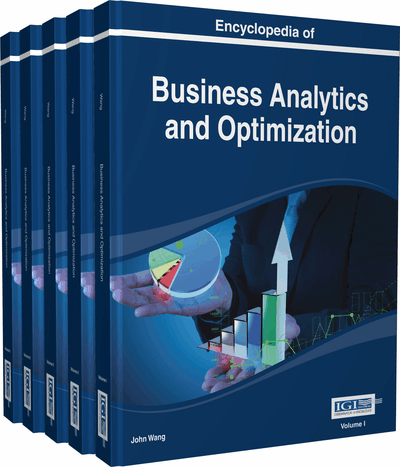# Bayesian Variable Selection

Oleg Okun (SMARTTECCO, Stockholm, Sweden)
DOI: 10.4018/978-1-4666-5202-6.ch023

Top

## Introduction

Variable selection is an important task in Predictive Analytics as it aims at eliminating redundant or irrelevant variables from a predictive model (either supervised or unsupervised) before this model is deployed in production. When the number of variables exceeds the number of instances, any predictive model will likely overfit the data, implying poor generalization to new, previously unseen instances. Even if for some data sets the number of variables is (much) smaller than the number of instances, some of collected variables may still harm the model performance if left in a data set because these variables may mask (hide) good for prediction variables. Therefore these “harmful” variables need to be discovered and removed.

There are hundreds techniques proposed for variable selection (see, for example, the book of (Liu & Motoda, 2008) entirely devoted to various variable selection methods). The purpose of this chapter is not to present as many of them as possible but concentrate on one type of algorithms, namely Bayesian variable selection (Lunn, Jackson, Best, Thomas, & Spiegelhalter, 2013). Again, as there are many such algorithms (see, for example, the survey in (O'Hara & Sillanpää, 2009)), we explain the general idea on the example of a particular representative algorithm described in this chapter.

Why Bayesian variable selection? Bayesian variable selection methods come equipped with measures of uncertainty, such as the posterior probability of each model and the variable importance specified by marginal inclusion probabilities. Model uncertainly can be incorporated into prediction through model averaging, which usually improves prediction. Missing data and/or non-Gaussian data distributions are easily handled by Markov Chain Monte Carlo (MCMC) simulations, which are the part of Bayesian variable selection.

Bayesian methods such as “stochastic search variable selection” (George & McCulloch, 1996) have been proposed as alternatives to traditional stepwise variable selection procedures in regression models. Instead of either fixing a regression coefficient at zero or allowing it to be estimated by least squares, as in stepwise procedures, stochastic search variable selection assigns a mixture prior distribution for the given coefficient. Both components of this prior are centered at zero but one with a small variance and the other with a large variance.

In general, there is a vector of regression coefficientsand a vectorof the same length containing 0/1 indicators, where 1 means a variable is included in a model and 0 implies that a variable is omitted from a model. The classical Bayesian variable selection (George & McCulloch, 1996) thus corresponds to the following model:

## Key Terms in this Chapter

Prior Distribution: A probability distribution that summarizes information about a random variable or parameter prior to obtaining further information from empirical data.

Probit Regression Model: A regression where the dependent variable only takes two values corresponding to two classes of data. Class membership for an observation or instance is decided based on the probability specified by the normal cumulative distribution function.

Bayesian variable selection: Selection of a subset of variables from the original set of variables based on Bayesian methods.

Latent Variable: A variable that cannot be measured directly, but is assumed to be related to one or more observable variables.

Markov Chain: A random process where the next state only depends on the current state but not on the states preceding the current state.

Posterior Distribution: A probability distribution that summarizes information about a random variable or parameter after obtaining new information from empirical data.

Burn-In Period: In Markov Chain Monte Carlo methods, the number of iterations needed before random samples from the specified distribution are taken into account.

Markov Chain Monte Carlo (MCMC): The class of random sampling methods from probability distributions based on Markov chain. A Gibbs sampler is one of the well known MCMC methods.

## Complete Chapter List

Search this Book:
Reset